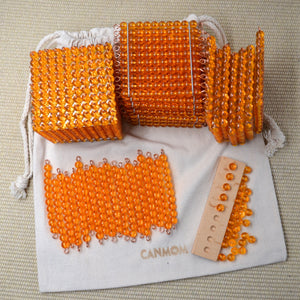# Montessori golden beads / Montessori decimal system

• \$88.00
Unit price per

This Montessori math set includes
1) 1 thousand cube
2) 18 hundreds
3) 18 tens bars
4) 50 units (you only need 18 units for work. the rest are for spare)
5) a base for 9 units (It only have 9 holes not 10 holes. When there is 10 units. those units need to exchange to ten bead bar)
6) FREE worksheets for place value (digital copy)

This Montessori toy set will be good for addition, subtraction, multiplication and division with CARRY or BORROW needed.

This Montessori material is used to introduce thousand, hundred, tens and units.

With this Montessori toy, a child won't get confuse with a long number
For example ,
number : 1432
Some children say that's 1,4,3,2
but it is 1 thousands 4 hundreds 3 tens and 2 units

It is one of my favourite Montessori material. We use it in preschool , kindergarten , grade 1, grand 2 and grade 3. It is a valuable materials as you can use for a long time.
We use it for the introduction of place value ( 1, 10, 100, 1000 ) addition, subtraction, multiplication and division.
For dynamic addition (for example 57+39), we need to CARRY. this material shows how we CARRY the beads physically! This visual experience solves a lot of confusion.

For dynamic subtraction (for example 77-29), we need to BORROW. This material shows us how we BORROW as well

***This material is very easy to understand and use. Give it a try and you will be surprise that 4 digits addition, subtraction , multiplication, division are so easy! ******

Math instruction
Step 1
Introduction of quality
With this concrete Montessori material, it gives children the visual experience of thousand, hundred, tens and unit.
A child knows that thousand is more than hundred by looking at the size

Teaching purpose:
- introduce the child to the concept of the decimal system and place value.
- make the child familiar with the names and relative sizes of the categories
- help the child with the difference in bulk between e.g. 6 units and 6 thousands.

Step 2
Introduction of symbols . learning numbers
Children match the golden beads materials to the corresponding number cards.

Teaching purpose:
acquaint the child with the written symbols for the quantities/categories of the decimal system.

Step3
Addition with decimal system. With this Montessori material, children move the two groups of beads together. They do the addition by combining the beads, not just learning the symbol of + on paper

Teaching purpose:
- give the impression of the nature of addition, that is of two or more small numbers coming together to form one larger number.